## Estimating Continuous-Time Models using Simulink Data

This example illustrates how models simulated in Simulink® can be identified using System Identification Toolbox™. The example describes how to deal with continuous-time systems and delays, as well as the importance of the intersample behavior of the input.

```if exist('start_simulink','file')~=2 disp('This example requires Simulink.') return end ```

### Acquiring Simulation Data from a Simulink Model

Consider the system described by the following Simulink model:

```open_system('iddemsl1') set_param('iddemsl1/Random Number','seed','0') ```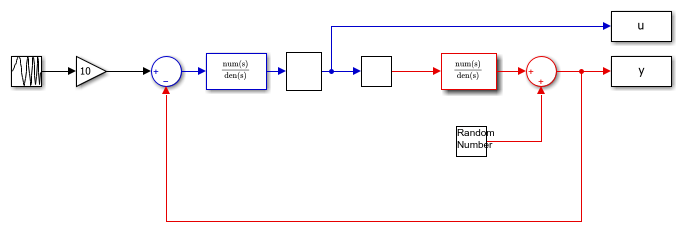The red part is the system, the blue part is the controller and the reference signal is a swept sinusoid (a chirp signal). The data sample time is set to 0.5 seconds.

This system can be represented using an `idpoly` structure:

```m0 = idpoly(1,0.1,1,1,[1 0.5],'Ts',0,'InputDelay',1,'NoiseVariance',0.01) ```
```m0 = Continuous-time OE model: y(t) = [B(s)/F(s)]u(t) + e(t) B(s) = 0.1 F(s) = s + 0.5 Input delays (listed by channel): 1 Parameterization: Polynomial orders: nb=1 nf=1 nk=0 Number of free coefficients: 2 Use "polydata", "getpvec", "getcov" for parameters and their uncertainties. Status: Created by direct construction or transformation. Not estimated. ```

Let us simulate the model `iddemsl1` and save the data in an `iddata` object:

```sim('iddemsl1') dat1e = iddata(y,u,0.5); % The IDDATA object ```

Let us do a second simulation of the mode for validation purposes.

```set_param('iddemsl1/Random Number','seed','13') sim('iddemsl1') dat1v = iddata(y,u,0.5); ```

Let us have a peek at the estimation data obtained during the first simulation:

```plot(dat1e) ```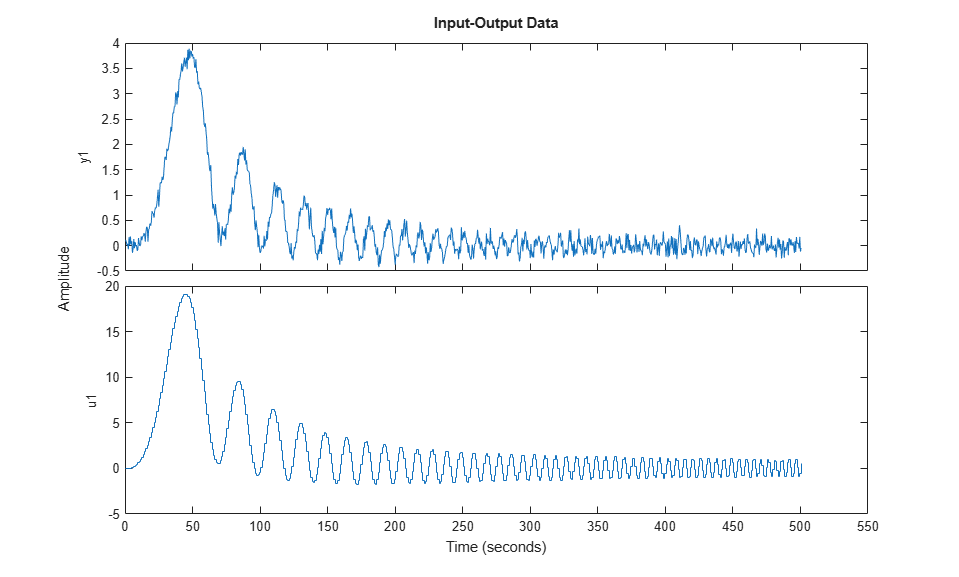### Estimating Discrete Models Using the Simulation Data

Let us begin by evaluating a default-order discrete model to gain some preliminary insight into the data characteristics:

```m1 = n4sid(dat1e, 'best') % A default order model ```
```m1 = Discrete-time identified state-space model: x(t+Ts) = A x(t) + B u(t) + K e(t) y(t) = C x(t) + D u(t) + e(t) A = x1 x2 x3 x1 0.7881 0.1643 0.1116 x2 -0.1214 0.4223 -0.8489 x3 0.155 0.7527 0.2119 B = u1 x1 -0.0006427 x2 -0.02218 x3 0.07347 C = x1 x2 x3 y1 -5.591 0.871 1.189 D = u1 y1 0 K = y1 x1 -0.001856 x2 0.002363 x3 -0.06805 Sample time: 0.5 seconds Parameterization: FREE form (all coefficients in A, B, C free). Feedthrough: none Disturbance component: estimate Number of free coefficients: 18 Use "idssdata", "getpvec", "getcov" for parameters and their uncertainties. Status: Estimated using N4SID on time domain data "dat1e". Fit to estimation data: 86.17% (prediction focus) FPE: 0.01281, MSE: 0.01251 ```

Check how well the model reproduces the validation data

```compare(dat1v,m1) ```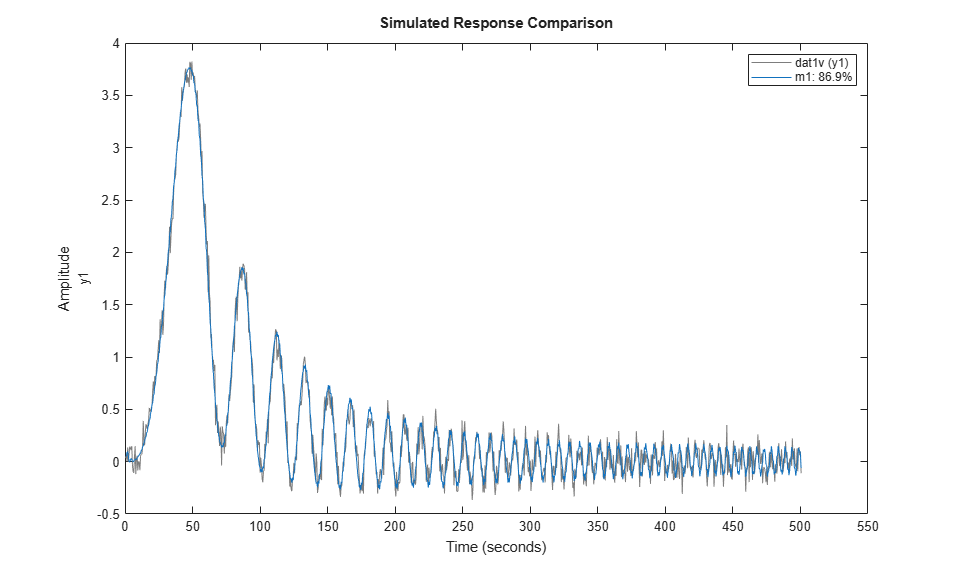As observed, the validation data is predicted well by the model. To investigate more into the data characteristics, let us inspect the non-parametric impulse response computed using `dat1e` where the negative lags for analysis are automatically determined:

```ImpModel = impulseest(dat1e,[],'negative'); clf h = impulseplot(ImpModel); showConfidence(h,3) ```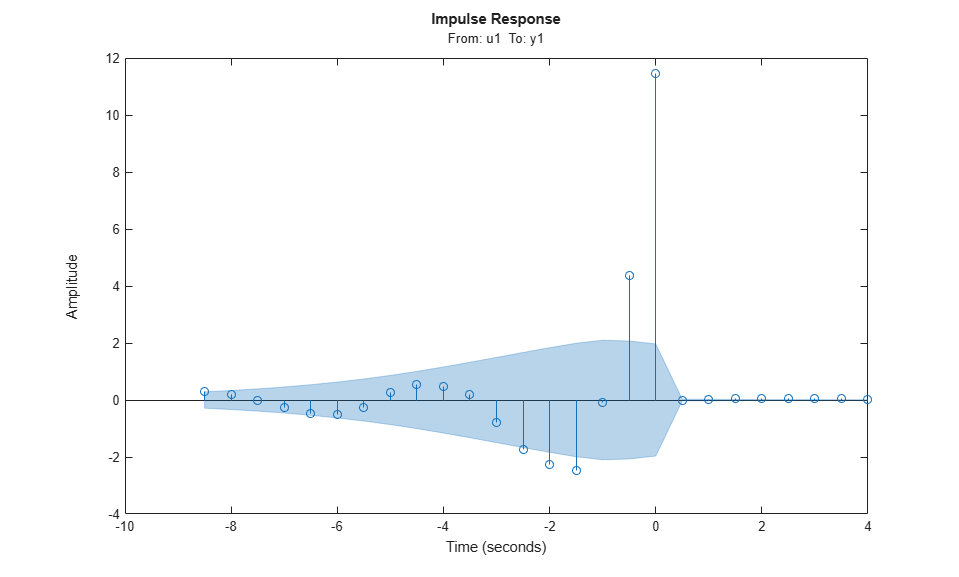`ImpModel` is an FIR model whose order (no. of coefficients) are automatically determined. We also choose to analyze feedback effects by computing impulse response for negative delays. Influences from negative lags are not all insignificant. This is due to the regulator (output feedback). This means that the impulse response estimate cannot be used to determine the time delay. Instead, build several low order ARX-models with different delays and find out the best fit:

```V = arxstruc(dat1e,dat1v,struc(1:2,1:2,1:10)); nn = selstruc(V,0) %delay is the third element of nn ```
```nn = 2 2 3 ```

The delay is determined to 3 lags. (This is correct: the deadtime of 1 second gives two lag-delays, and the ZOH-block another one.) The corresponding ARX-model can also be computed, as follows:

```m2 = arx(dat1e,nn) compare(dat1v,m1,m2); ```
```m2 = Discrete-time ARX model: A(z)y(t) = B(z)u(t) + e(t) A(z) = 1 - 0.2568 z^-1 - 0.3372 z^-2 B(z) = 0.04021 z^-3 + 0.04022 z^-4 Sample time: 0.5 seconds Parameterization: Polynomial orders: na=2 nb=2 nk=3 Number of free coefficients: 4 Use "polydata", "getpvec", "getcov" for parameters and their uncertainties. Status: Estimated using ARX on time domain data "dat1e". Fit to estimation data: 85.73% (prediction focus) FPE: 0.01346, MSE: 0.0133 ```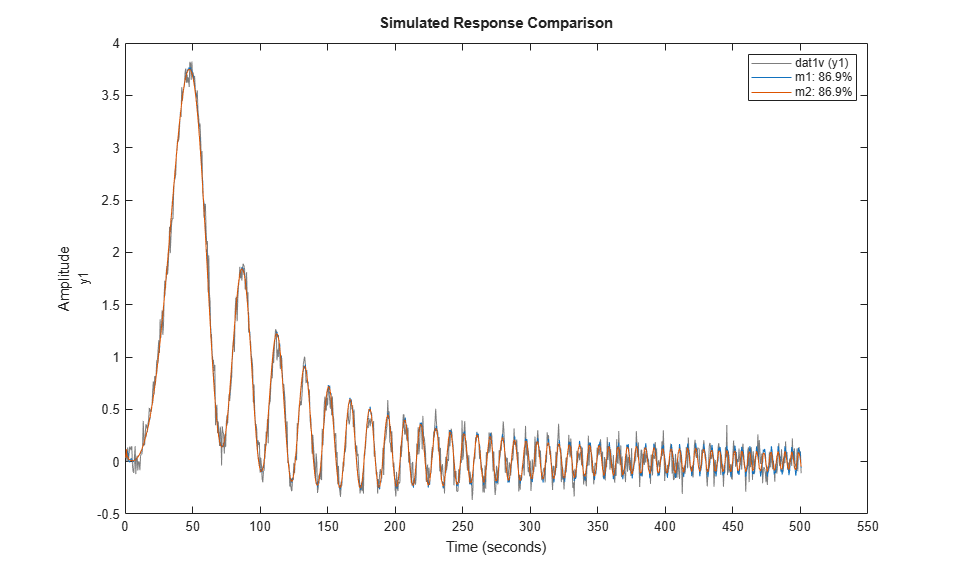### Refining the Estimation

The two models `m1` and `m2` behave similarly in simulation. Let us now try and fine-tune orders and delays. Fix the delay to 2 (which coupled with a lack of feedthrough gives a net delay of 3 samples) and find a default order state-space model with that delay:

```m3 = n4sid(dat1e,'best','InputDelay',2,'Feedthrough',false); % Refinement for prediction error minimization using pem (could also use % |ssest|) m3 = pem(dat1e, m3); ```

Let as look at the estimated system matrix

```m3.a % the A-matrix of the resulting model ```
```ans = 0.7332 -0.3784 0.1735 0.4705 0.3137 -0.6955 -0.0267 0.7527 0.6343 ```

A third order dynamics is automatically chosen, which together with the 2 "extra" delays gives a 5th order state space model.

It is always advisable not to blindly rely upon automatic order choices. They are influenced by random errors. A good way is to look at the model's zeros and poles, along with confidence regions:

```clf h = iopzplot(m3); showConfidence(h,2) % Confidence region corresponding to 2 standard deviations ```Clearly the two poles/zeros at the unit circle seem to cancel, indicating that a first order dynamics might be sufficient. Using this information, let us do a new first-order estimation:

```m4 = ssest(dat1e,1,'Feedthrough',false,'InputDelay',2,'Ts',dat1e.Ts); compare(dat1v,m4,m3,m1) ```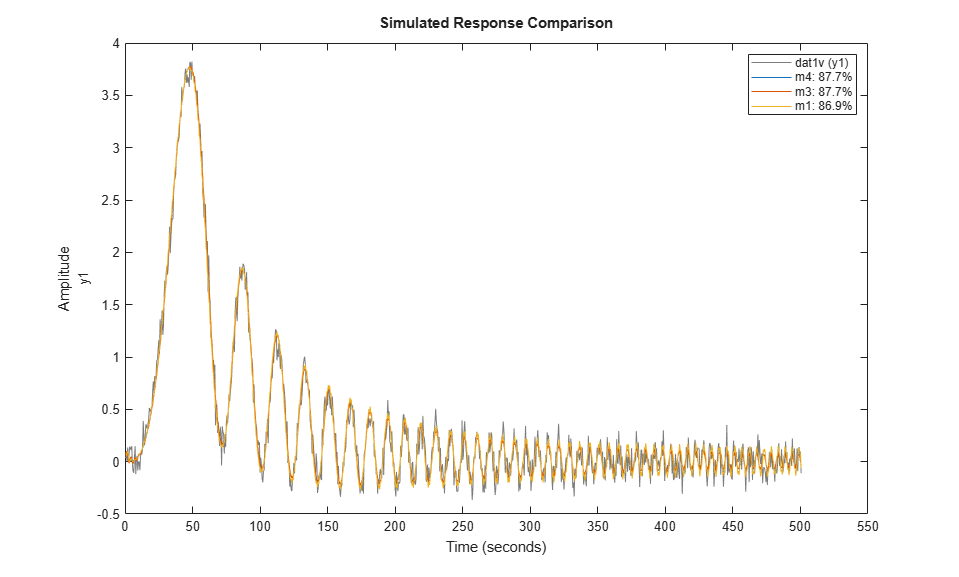The `compare` plot shows that the simple first order model `m4` gives a very good description of the data. Thus we shall select this model as our final result.

### Converting Discrete Model to Continuous-Time (LTI)

Convert this model to continuous time, and represent it in transfer function form:

```mc = d2c(m4); idtf(mc) ```
```ans = From input "u1" to output "y1": 0.09828 exp(-1*s) * ---------- s + 0.4903 Continuous-time identified transfer function. Parameterization: Number of poles: 1 Number of zeros: 0 Number of free coefficients: 2 Use "tfdata", "getpvec", "getcov" for parameters and their uncertainties. Status: Created by direct construction or transformation. Not estimated. ```

A good description of the system has been obtained, as displayed above.

### Estimating Continuous-Time Model Directly

The continuous time model can also be estimated directly. The discrete model `m4` has 2 sample input delay which represents a 1 second delay. We use the `ssest` command for this estimation:

```m5 = ssest(dat1e,1,'Feedthrough',false,'InputDelay',1); present(m5) ```
``` m5 = Continuous-time identified state-space model: dx/dt = A x(t) + B u(t) + K e(t) y(t) = C x(t) + D u(t) + e(t) A = x1 x1 -0.4903 +/- 0.007881 B = u1 x1 0.01345 +/- 6.728e+10 C = x1 y1 7.307 +/- 3.655e+13 D = u1 y1 0 K = y1 x1 -0.02227 +/- 1.114e+11 Input delays (seconds): 1 Parameterization: FREE form (all coefficients in A, B, C free). Feedthrough: none Disturbance component: estimate Number of free coefficients: 4 Use "idssdata", "getpvec", "getcov" for parameters and their uncertainties. Status: Termination condition: No improvement along the search direction with line search.. Number of iterations: 9, Number of function evaluations: 152 Estimated using SSEST on time domain data "dat1e". Fit to estimation data: 87.34% (prediction focus) FPE: 0.01054, MSE: 0.01047 More information in model's "Report" property. ```

### Uncertainty Analysis

The parameters of model `m5` exhibit high levels of uncertainty even though the model fits the data 87%. This is because the model uses more parameters than absolutely required leading to a loss of uniqueness in parameter estimates. To view the true effect of uncertainty in the model, there are two possible approaches:

1. View the uncertainty as confidence bounds on model's response rather than on the parameters.

2. Estimate the model in canonical form.

Let use try both approaches. First we estimate the model in canonical form.

```m5Canon = ssest(dat1e,1,'Feedthrough',false,'InputDelay',1,'Form','canonical'); present(m5Canon) ```
``` m5Canon = Continuous-time identified state-space model: dx/dt = A x(t) + B u(t) + K e(t) y(t) = C x(t) + D u(t) + e(t) A = x1 x1 -0.4903 +/- 0.007881 B = u1 x1 0.09828 +/- 0.001559 C = x1 y1 1 D = u1 y1 0 K = y1 x1 -0.1628 +/- 0.03702 Input delays (seconds): 1 Parameterization: CANONICAL form with indices: 1. Feedthrough: none Disturbance component: estimate Number of free coefficients: 3 Use "idssdata", "getpvec", "getcov" for parameters and their uncertainties. Status: Termination condition: No improvement along the search direction with line search.. Number of iterations: 9, Number of function evaluations: 152 Estimated using SSEST on time domain data "dat1e". Fit to estimation data: 87.34% (prediction focus) FPE: 0.01054, MSE: 0.01047 More information in model's "Report" property. ```

`m5Canon` uses a canonical parameterization of the model. It fits the estimation data as good as the model `m5`. It shows small uncertainties in the values of its parameters giving an evidence of its reliability. However, as we saw for `m5`, a large uncertainty does not necessarily mean a "bad" model. To ascertain the quality of these models, let use view their responses in time and frequency domains with confidence regions corresponding to 3 standard deviations. We also plot the original system `m0` for comparison.

The bode plot.

```clf opt = bodeoptions; opt.FreqScale = 'linear'; h = bodeplot(m0,m5,m5Canon,opt); showConfidence(h,3) legend show ```The step plot.

```clf showConfidence(stepplot(m0,m5,m5Canon),3) legend show ```The uncertainty bounds for the two models are virtually identical. We can similarly generate pole-zero map (`iopzplot`) and Nyquist plot (`nyquistplot`) with confidence regions for these models.

```idtf(m5) ```
```ans = From input "u1" to output "y1": 0.09828 exp(-1*s) * ---------- s + 0.4903 Continuous-time identified transfer function. Parameterization: Number of poles: 1 Number of zeros: 0 Number of free coefficients: 2 Use "tfdata", "getpvec", "getcov" for parameters and their uncertainties. Status: Created by conversion from idss model. ```

### Accounting for Intersample Behavior in Continuous-Time Estimation

When comparing continuous time models computed from sampled data, it is important to consider the intersample behavior of the input signal. In the example so far, the input to the system was piece-wise constant, due to the Zero-order-Hold (zoh) circuit in the controller. Now remove this circuit, and consider a truly continuous system. The input and output signals are still sampled a 2 Hz, and everything else is the same:

```open_system('iddemsl3') sim('iddemsl3') dat2e = iddata(y,u,0.5); ```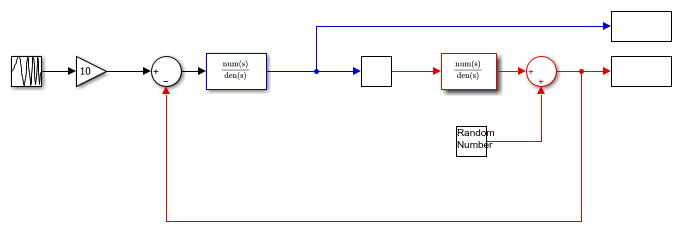Discrete time models will still do well on these data, since when they are adjusted to the measurements, they will incorporate the sampling properties, and intersample input behavior (for the current input). However, when building continuous time models, knowing the intersample properties is essential. First build a model just as for the ZOH case:

```m6 = ssest(dat2e,1,'Feedthrough',false,'InputDelay',1,'Form','canonical'); idtf(m6) ```
```ans = From input "u1" to output "y1": 0.1117 exp(-1*s) * ---------- s + 0.5596 Continuous-time identified transfer function. Parameterization: Number of poles: 1 Number of zeros: 0 Number of free coefficients: 2 Use "tfdata", "getpvec", "getcov" for parameters and their uncertainties. Status: Created by conversion from idss model. ```

Let us compare the estimated model (`m6`) against the true model (`m0`):

```step(m6,m0) % Compare with true system ```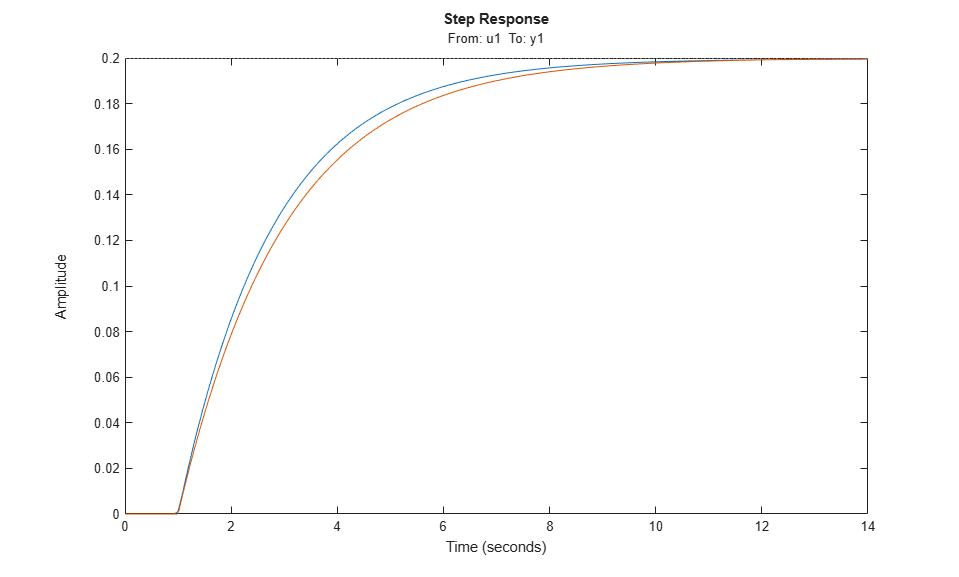The agreement is now not so good. We may, however, include in the data object information about the input. As an approximation, let it be described as piecewise linear (First-order-hold, FOH) between the sampling instants. This information is then used by the estimator for proper sampling:

```dat2e.Intersample = 'foh'; m7 = ssest(dat2e,1,'Feedthrough',false,'InputDelay',1,'Form','canonical'); % new estimation with correct intersample behavior idtf(m7) ```
```ans = From input "u1" to output "y1": 0.09937 exp(-1*s) * ---------- s + 0.4957 Continuous-time identified transfer function. Parameterization: Number of poles: 1 Number of zeros: 0 Number of free coefficients: 2 Use "tfdata", "getpvec", "getcov" for parameters and their uncertainties. Status: Created by conversion from idss model. ```

Let us look at the step response comparison again:

```step(m7,m0) % Compare with true system ```This model (`m7`) gives a much better result than `m6`. This concludes this example.

```bdclose('iddemsl1'); bdclose('iddemsl3'); ```
##### SupportGet trial now